# Page History

Search TestNav 8 Online Support:

## Key

• This line was removed.
• Formatting was changed.

...

TestNav ToolbarToolAllows students to:

Pointer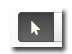Close any enabled tools.

Quarter-inch Ruler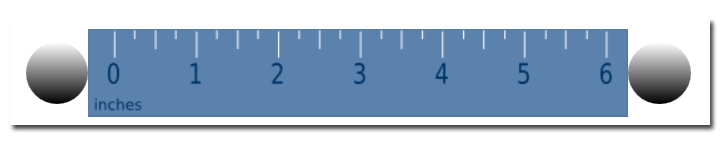Measure lengths in ¼-inch increments.

Eighth-inch Ruler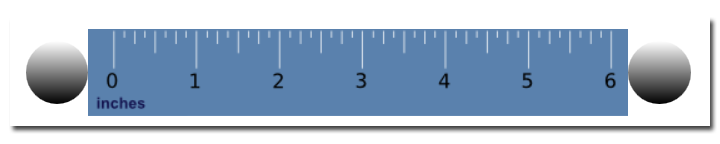Measure lengths in ⅛-inch increments.

Back to toolbar

Protractor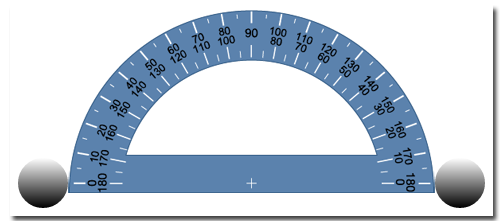Measure angles, and drag and rotate the protractor.

Back to toolbar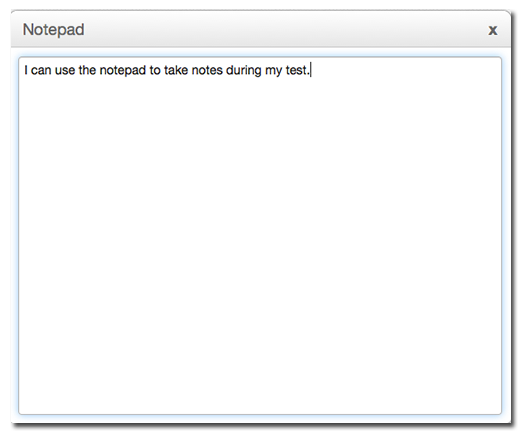Type notes while testing.

Four-function Calculator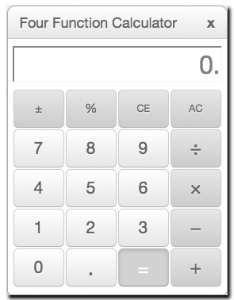Perform four functions:

• Subtraction
• Division
• Multiplication

Five-function Calculator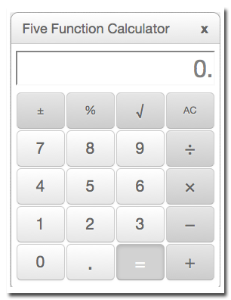Perform five functions:

• Subtraction
• Division
• Multiplication
• Square root

Back to toolbar

Centimeter Ruler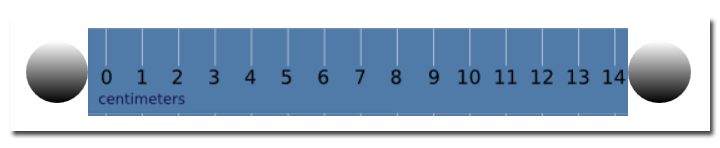Measure length in 1-cm increments.

Back to toolbar

Scientific Calculator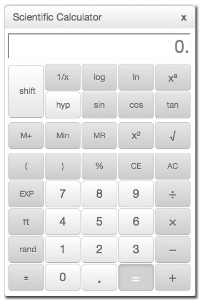Perform a range of functionality including:

• General math
• Algebra
• Trigonometry
• Statistics

Back to toolbar

TI-84 Graphing Calculator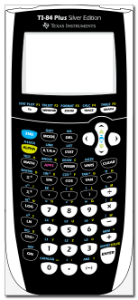Perform a range of functionality including:

• General math
• Algebra
• Trigonometry
• Statistics
• Graphing

Back to toolbarVisually eliminate (with a red X) one or more answers.

Back to toolbar

TI-108 Basic Calculator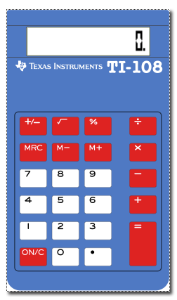Perform basic arithmetic functions:

• Subtraction
• Division
• Multiplication

Back to toolbar

TI-30 Scientific Calculator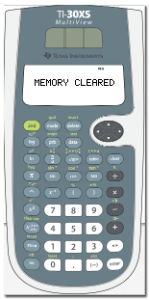Perform high school math and science on four lines.

Back to toolbar

...